## Chapter 4Statistics

### 4.1 Why do I need to know statistics?

There will be lots of cases where you’ll need to know some basic statistical concepts as a recording engineer. For example, you may have already seen a meter on a mixing console that tells you the correlation of your left and right channels. To know what this means, you’ll need a little background in statistics. Read a paper on multichannel microphone technique and you’ll see coherence mentioned. More stats. Even an RMS voltage measurement is another name for a statistical idea called the standard deviation (sort of...).

If you’re doing DSP or working on digital hardware, you’ll need to have a thorough understanding of dither, which, in turn means that you’ll have to have a firm grasp of the concept of a probability distribution function.

Of course, if you’re more interested in things like listening tests, or if you read papers that talk about such things, you’ll definitely need to know a thing or two about concepts like a mean, standard deviation and a confidence interval.

### 4.2 Population

The population is the collection of all of your measurements. Let’s say that you’re doing a statistical analysis on the ages of a classroom of thirty grade 10 students. In this case, the population is the list of thirty ages.

It’s comprised of N measurements, each of which is labeled xi (the i refers to the index number of the measurement)

One thing to remember is that a population is a collection of measurements – not the collection of things being measured. So, if we’re looking at the ages of grade 10 students, it’s the list of ages that’s the population, not the students themselves.

### 4.3 Sample

A sample in statistics is a subset of the population. If we looked at the ages of five of the thirty students in the class mentioned above, we’d be using a sample of the population.

It’s comprised of n measurements, each of which is labeled xi (the i refers to the index number of the measurement)

Samples are often used in statistics because you can’t know everything about everybody. For example, if we want to know something about the ages of all grade 10 students in Canada, we could find out the actual age of every grade student in Canada (the population) or we could just look at the grade 10 classes in a couple of schools (a sample) and hope that the rest of the population has similar characteristics.

### 4.4 Mean

The (mean) of a given population is what we normal people usually call the average.

There are two types of means:

#### 4.4.1 Population mean

abbreviated m

Population mean =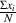(In other words, it’s the sum of all the measurements in the population divided by the total number of measurements.)

#### 4.4.2 Sample mean

abbreviated X

X =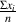Calculate this the same way, but only use the measurements from the sample, and the total number of measurements in the sample.

### 4.5 Median

The median, abbreviated M, is the the value in the middle position of your population or sample when the measurements are ordered from smallest to largest.

For example, if your measurements are 3, 6, 4, 8, and 5, then you re-order them, 3, 4, 5, 6, 8 and take the middle one: 5

However, if your measurements are 3, 6, 8, and 5, then you re-order them, 3, 5, 6, 8, and take the half-way point between the two middle ones (5+6)/2 = 5.5

### 4.6 Mode

The category that occurs most frequently. The class with the highest frequency is called the modal class.

For example, if your measurements are 3, 6, 4, 5, 8, 4, 8, 6, 8, and 9, then the mode is 8 because it appeared three times – more frequently than any other number.

### 4.7 Range

The range, abbreviated R, is the difference between the largest and the smallest measurements in your population or sample.

### 4.8 Deviation

The difference between a measurement and the mean.

This is also called the deviation from the mean or the residual.

### 4.9 Variance

the variance of a population of N measurements is denoted s2.

This is the mean of the squares of the deviations of the measurements.

s2 =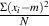The variance of a sample of n measurements is denoted s2

This is the sum of the squared deviations of the measurements about the sample mean divided by (n-1)

CHECK THIS – SHOULDN’T IT BE n AND NOT n-1?

### 4.10 Standard deviation

The standard deviation, abbreviated s, is the square root of the variance

s =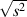It’s a calculation of the variability of your measurements which, happily, is in the same units as the measurements themselves.

Note that, when the mean is 0 (as it is in the case of most audio signals), the standard deviation is the same as the RMS value of the signal.

### 4.11 Standard error

a value which indicates “how well the sample average estimates the population mean”

standard error of the average =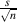Note the relationship between the sample size and the standard error: in order to double your precision, you need to quadruple your sample size.

### 4.12 Percentile

1. Put your measurements in order from smallest to largest

2. the pth percentile is the value of x that exceeds p% of the measurements and is less than (100-p)%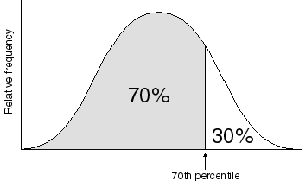Figure 4.1: A graphical representation of the 70th percentile in a standard distribution of values.

The 25th percentile is also known as the lower quartile, first quartile or Q1.

The 50th percentile is also known as the mean

The median is the second quartile or Q2

The 75th percentile is also known as the upper quartile, third quartile or Q3

The Interquartile range is Q3 -Q1

### 4.13 c2 or Chi-Square distribution

(say “key” – it’s Greek)

This is a value used to determine the independence of two things. You’ll need to know what you’re expecting from your data and what you actually get as results to make this calculation. For example: let’s say you wanted to do an experiment where you were trying to find out if the orientation of a coin on your thumb before you tossed it had any effect on whether it lands heads or tails. You toss the coin 50 times starting heads up, and 50 times tails up and take note of how it lands and write it all down. You’re also going to write down what you expect – half of the time it should land heads up and half the time tails up, no matter what the orientation before we make the throw...

 Pre-throw Lands Lands Expected Expected orientation “Heads” “Tails” “Heads” “Tails” Heads 29 21 25 25 Tails 19 31 25 25

Table 4.1: INSERT CAPTION HERE

Calculating the Chi-Square Statistic:

c2 = S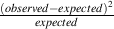The bigger this number is, the more different the actual measurements are from the values you expected to get.

After that, you find the degrees of freedom which is one less than the number of categories.

All of this can be done most easily using software. Pretty well any spreadsheet will look after it – look for a function named CHITEST. This will probably give you a small number which has to be converted into the chi-square statistic. Then you use a function named something like CHIINV.

If you go through the math, you’ll find that the results of the CHITEST for the above table is about 0.0414. The degree of freedom in this example is 1 (number of rows – 1)*(number of columns – 1). When you put those numbers in a CHIINV, you get 4.16.

Once you have those numbers, you’ll need to do some digging through a stats book to find a chi-square table. This will list the critical values for a number of percentages and degrees of freedom. For example, if I look up the 5% critical value for 1 degree of freedom, I find out that the value is 3.841. You have to compare this number (3.841) with the number you get (in our example, 4.16). If your value is bigger than the one in the table, then that means that your actual values are significantly different than what you expected, and that the two categories are NOT independent. If your calculated value is smaller than the one in the table, then we can say that any variations in the answers you got are within the acceptable limits associated with random things that happened in the test.

Note, however, that the critical value is associated with a percentage level – in this case 5% (or 0.05). This tells you the number of times out of a hundred that you’re going to be wrong if you use this data to predict that the whole population will give you the same results.

### 4.14 Correlation Coefficient

The term “correlation” is one that is frequently misused and, as a result, misunderstood in the field of audio engineering. Consequently, some discussion is required to define the term. Generally speaking, the correlation of two audio signals is a measure of the relationship of these signals in the time domain . Specifically, given two two-dimensional variables (in the case of audio, the two dimensions are amplitude and time), their correlation coefficient, r is calculated using their covariance sxy and their standard deviations sx and sy as is shown in Equation 4.1 . The line over these three components indicates a time average, as is discussed below.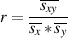(4.1)

The standard deviation of a series of values is an indication of the average amount the individual values are different from the total average for all values. Specifically, it is the square root of the average of the squares of the differences between the average of all values and each individual value. For example, in order to find the standard deviation of a PCM digital audio signal, we begin by finding the average of all sample values. This will likely be 0 since audio signals typically do not contain a DC offset. Each sample is then individually subtracted from this average and each result is squared. The average of these squares is calculated and its square root is the standard deviation. When there is no DC component in an audio signal, its standard deviation is equal to its RMS value. In such a situation, it can be considered the square root of the average power of the signal.

The covariance of two series of values is an indication of whether they are interrelated. For example, if the average temperature for today’s date is 19 C and the average humidity is 50%, yet today’s actual temperature and humidity are 22 C and 65%, we can find whether there is an interdependent relationship between these two values, called the covariation . This is accomplished by multiplying the differences of today’s values from their respective averages, therefore (19 – 22) * (50 – 65) = 45. The result of this particular calculation, being a positive number, indicates that there is a positive relationship between the temperature and humidity today – in other words, if one goes up, the other does also. Had the covariation been negative, then the relationship would indicate that the two variables had behaved oppositely. If the result is 0, then at least one of the variables equalled the average value. The covariance is the average of the covariations of two variables measured over a period of time. The difficulty with this measurement is that its scale changes according to the scale of the two variables being measured. Consequently, covariance values for different statistical samples cannot be compared. For example, we cannot tell whether the covariance of air temperature and humidity is greater or less than the covariance of the left and right channels in a stereo audio recording if both have the same polarity.

Fortunately, if the standard deviations of the two signals are multiplied, the scale is identical to that of the covariance. Therefore, the correlation coefficient (the covariance divided by the product of the two standard deviations) can be considered to be a normalised covariance. The result is a value that can range from -1 to 1 where 1 indicates that the two signals have a positive linear relationship (in other words, they always have the same polarity if we remove any DC offset they might have). A correlation coefficient of -1 indicates that the two signals are negatively linearly related (therefore, they always have opposite polarities when we remove their DC offsets). In the case of wide-band signals, a correlation of 0 usually indicates that the two signals are either completely unrelated or separated in time by a delay greater than the averaging time.

In the particular case of two sinusoidal waveforms with identical frequency and a constant phase difference ωτ, Equation 4.1 can be simplified to Equation 4.2 .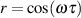(4.2)

where the radian frequency ω is defined in Equation 4.3 and where τ is the time separation of the two sinusoids.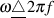(4.3)

where the symbol denotes “is defined as” and f is the frequency in Hz.

Further investigation of the topic of correlation highlights a number of interesting points. Firstly, two signals of identical frequency and phase have a correlation of 1. It is important to remember that this is true regardless of the amplitudes of the two signals [Welle, ]. Two signals of identical frequency and with a phase difference of 180 have a correlation of -1. Signals of identical frequency and with a phase difference of ±90 have a correlation of 0. Finally, it is important to remember that the correlation between two signals is highly dependent on the amount of time used in the averaging process.

### 4.15 Coherence

In order to understand the concept of coherence, we need to discuss a couple of other things first. As a result, this topic is discussed later in the book in the chapter on DSP.

### 4.16 Probability Distribution Functions

Flip a coin. You’ll get a heads or a tails. Flip it again, and again and again, each time writing down the result. If you flipped the coin 1000 times, chances are that you’ll see that you got a heads about 500 times and a tails about 500 times. This is because each side of the coin has an equal chance of landing up, therefore there is a 50% probability of getting a heads, and a 50% probability of getting a tails. If we were to draw a graph of this relationship, with “heads” and “tails” being on the x-axis and the probability on the y-axis, we would have two points, both at 50%.

Let’s do basically the same thing by rolling a die. If we roll it 600 times, we’ll probably see around 100 1’s, 100 2’s, 100 3’s, 100 4’s, 100 5’s and 100 6’s. Like the coin, this is because each number has an equal probability of being rolled. I tried this, and kept track of each number that was rolled an got the numbers shown in Table 4.2. If we were to graph this information, it would look like Figure 4.2.

 Result 1 2 3 4 5 6 Number of 111 98 101 94 92 104 times rolled

Table 4.2: The results of rolling a die 600 times.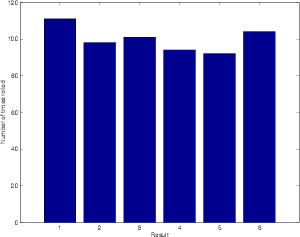Figure 4.2: The results of 600 rolls of a die. This is a plot of the information shown in Table 4.2.

Let’s say that we didn’t know that there was an equal probability of rolling each number on the die. How could we find this out experimentally? All we have to do is to take the numbers in Table 4.2 and divide by the number of times we rolled the die. This then tells us the probability (or the chances) of rolling each number. If the probability of rolling a number is 1, then it will be rolled every time. If the probability is 0, then it will never be rolled. If it is 0.5, then the number will be rolled half of the time.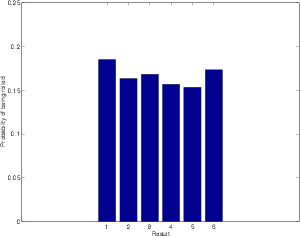Figure 4.3: The calculated probability of rolling each number on the die using the results shown in Table 4.2.

Notice that the numbers didn’t work out perfectly in this example, but they did come close. I was expecting to get each number 100 times, but there was a small deviation from this. The more times I roll the dice, the more reality will approach the theoretical expectation. To check this out, I did a second experiment where I rolled the die 60,000 times.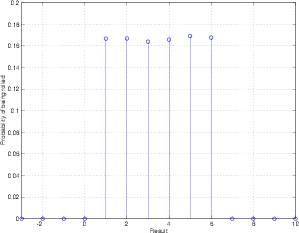Figure 4.4: The calculated probability of rolling each number on the die using the results after 60,000 rolls. Notice that the graph has a rectangular shape.

This graph tells us a number of things. Firstly, we can see that there is a 0 probability of rolling a 7 (this is obvious because there is no “7” on a die, so we can never roll and get that result). Secondly, we can see that there is an almost exactly equal probability of rolling the numbers from 1 to 6 inclusive. Finally, if we look at the shape of this graph, we can see that it makes a rectangle. So, we can say that rolling a die results in a rectangular probability density function or RPDF.

It’s possible to have different probability density functions. For example, what would happen if we rolled two dice? Let’s do it and find out. I rolled a pair of dice 600 times and kept track of the results. These are all listed in Table 4.3.

 Result Number of times rolled 2 21 3 36 4 55 5 72 6 78 7 87 8 84 9 60 10 50 11 37 12 20

Table 4.3: The results of rolling two dice 600 times.

Notice that I only rolled a “2” 21 times, but I rolled a “7” 87 times. This is because there was only one way that I could roll a 2 – by getting two 1’s. However, there are different ways to get a 7. There’s 1+6, 2+5, 3+4, 4+3, 5+2 and 6+1. So, it makes sense that I rolled a 7 four times as often as a 2 because there are four times as many combinations that result in a 7 than can result in a 2.

If I graph the results of the 600 rolls, we get the plot shown in Figure 4.5. Notice that it looks a bit like a triangle.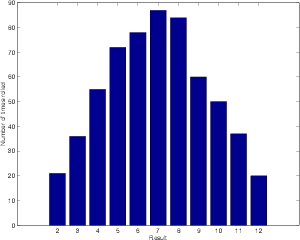Figure 4.5: The results of rolling two dice 600 times(this is a graph of the same data shown in Table 4.3).

If I do the same thing, but roll the pair of dice 60,000 times instead, we get something like the numbers shown in Figure 4.4.

 Result Number of times rolled 2 1657 3 3283 4 4935 5 6663 6 8430 7 9988 8 8368 9 6699 10 4996 11 3336 12 1645

Table 4.4: The results of rolling two dice 60000 times.

A graph of these numbers is shown in Figure 4.6 and the same graph represented as a probability (instead of the number of times the values were rolled) is shown in Figure 4.7. Notice that, when we roll so mane times, the graph really does look like a triangle. Consequently, we call it a triangular probability distribution function or TPDF.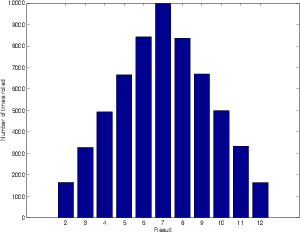Figure 4.6: results of rolling two dice 60000 times (this is a graph of the data shown in Table 4.4).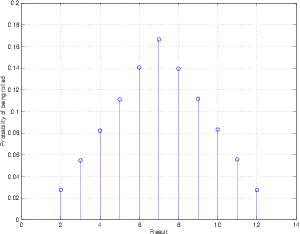Figure 4.7: The calculated probability of rolling each number on the two dice using the results shown in Table 4.3.

Let’s look at another probability density function. Let’s look at the ages of children in Grade 5. If we were to take all the Grade 5 students in Canada, ask them their age, and make a PDF out of the results, it might look like Figure 4.8.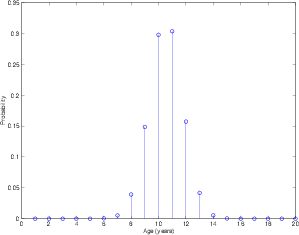Figure 4.8: The calculated probability of the age of students in Grade 5 in Canada.

This is obviously not an RPDF or a TPDF because the result doesn’t look like a rectangle or a triangle. In fact, it is what statisticians call a normal distribution, better known as a bell curve. What this tells us is that the probability a Canadian Grade 5 student of being either 10 or 11 years old is higher than for being any other age. It is possible, but less likely that the student will be 8, 9, 12 or 13 years old. It is extremely unlikely, but also possible for the student to be 7 or 14 years old.